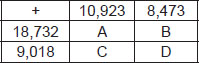# User ForumSubject :IMO    Class : Class 5

Complete the addition square by finding the missing numbers.
Find (A - B) - (C - D).A 0
B 450
C 1000
D 300

## Ans 1:

Class : Class 7
why? i did not understand

## Ans 2:

Class : Class 6
From the given table, we have A = 18,732 10,923 = 29,655 B = 18,732 8,473 = 27,205 C = 10,923 9,018 = 19,941 and D = 9,018 8,473 = 17,491 Now, A - B = 29,655 - 27,205 = 2,450 C - D = 19,941 - 17,491 = 2,450 â´ (A - B) - (C - D) = 2,450 - 2,450 = 0.

Class : Class 6
a 0

Class : Class 1
It is A i.e 0

Class : Class 5

## Ans 6:

Class : Class 6
Yes,0 is the answer

Class : Class 7
0 is the answer

## Ans 8:

Class : Class 7
See,deepak if 2-2 =0 ,then if the minued and the subtrahend are equal, the diff-erence is 0.

## Ans 9:

Class : Class 7
2450 - 2450 = 0 so the answer is .......................... A

## Ans 10:

Class : Class 1
not possible question

## Ans 11:

Class : Class 7
The answer is A:0if you write the number sentence (given:(A-B)-(C-D)),each number will add and subtract itself(without the bracket):10923 18732 - 8473 - 18732 - 10923 - 9018 8473 9018from this we can say that the answer is 0.

Class : Class 6

Class : Class 5

Class : Class 6

Class : Class 7
0

Class : Class 6
A) 0.